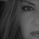# Short-term Volume And Price Oscillator [LazyBear]

Dilihat 8466 kali
8466
Short-term Volume and Price Oscillator (SVAPO), developed by Sylvian Vervroot, combines both Price and Volume to construct an oscillator. In essence, when the price is trending up and volume is increasing, volume is added into the oscillator calculation. Conversely, when price is trending down and volume is increasing, volume will be subtracted from the oscillator. During consolidation phases when price and volume diverge, volume is not used to calculate the oscillator.

Some notes from his book:
- A buy is indicated when the oscillator is below the green line but greater than yesterday’s value.
A sell is indicated when the oscillator is above the red line but less than yesterday’s value.
- The start of a short term up move is signaled by SVAPO when it turns up from below the lower standard
deviation boundary. The same is valid for a short term down move when SVAPO turns down from above the
upper standard deviation boundary.
- Medium term turning points in an up or downtrend are mostly announced with a divergence between price and
SVAPO. In a medium term uptrend, SVAPO will generally continue to move above the 0-reference line.

http://stocata.org/ta_en/proprietary.htm...

Vervroot sometimes uses this with his modified %B oscillator (https://www.tradingview.com/v/sDPe1PDd/).

List of my other indicators:
- Chart:
```//
// @author LazyBear
// List of all my indicators:
//
study("Short-term Volume And Price Oscillator [LazyBear]", shorttitle="SVAPO_LB")
length=input(8, title="SVAPO Period", minval=2, maxval=20)
cutoff=input(1, title="Minimum %o price change", maxval=10, minval=0)
devH=input(1.5, title="Stdev High", maxval=5, minval=0.1)
devL=input(1.3, title="Stdev Low", maxval=5, minval=0.1)
stdevper=input(100, title="Stdev Period", maxval=200, minval=1)

calc_tema(s, length) =>
ema1 = ema(s, length)
ema2 = ema(ema1, length)
ema3 = ema(ema2, length)
3 * (ema1 - ema2) + ema3

calc_linregslope(C, tp) =>
((tp*(sum(cum(1)*C,tp)))-(sum(cum(1),tp)*(sum(C,tp))))/((tp*sum(pow(cum(1),2),tp))-pow(sum(cum(1),tp),2))

calc_OR2(x) =>
y=x // To force expr evaluation
(y == true) or (y == true)

haOpen=(ohlc4 + nz(haOpen))/2
haCl=(ohlc4+haOpen+max(high, haOpen)+min(low, haOpen))/4
haC=calc_tema(haCl, round(length/1.6))
vma=sma(volume, length*5)
vave=vma
vmax=2*vave
vc=iff(volume<vmax, volume, vmax)
vtr=calc_tema(calc_linregslope(volume, length), length)
svapo=calc_tema(sum(iff(haC>(nz(haC)*(1+cutoff/1000)) and  calc_OR2((vtr>=nz(vtr))), vc, iff(haC<(nz(haC)*(1-cutoff/1000)) and calc_OR2((vtr>nz(vtr))),-vc,0)), length)/(vave+1),length)
plot(devH*stdev(svapo,stdevper), color=red, style=3)
plot(-devL*stdev(svapo,stdevper), color=green, style=3)
plot(0, color=gray, style=3)
plot(svapo, color=maroon, linewidth=2)```On shorter time frame (4h) or longer (weekly) seems to be annoying and needs to be used with extra tools for confirmation of reversals. While price is running into upper band (127%) and SVAPO displays oversold and a timid divergence- price continues the move up to 162%. So, one would be tempted to sell at the 127% because everything is pointing to a reversal and missing the last part of the impulse move. With some MAs (emas) this could be filtered or other indicators.
BalasVery nice tool LB- as usual great work. I gave it a test drive and seems to me that it showing more than expected. It looks very sensitive and one should analyse it also as a A/D tool too.

Now an image with the LazyBear COG a bit changed by me:) showing it is working also visually much better with it. The price making a dbl.top and the second top is below the red band also looking at the SVAPO position being in oversold teritory could lead to the conclusion that a distribution is coming. The AAPL price at the moment shows a bullish moment but the SVAPO shows a weakening of the recent impulse move. I would have liked the COG to have possibility of offsetting the bands 2-4 levels up and down.
Balassublimares2
Very interesting. Thanks for sharing, sublimares.
BalasRate of Change does make a difference in future price action with this indicator
BalasLazyBear
Looks like we can also see a band squeeze too
Balas